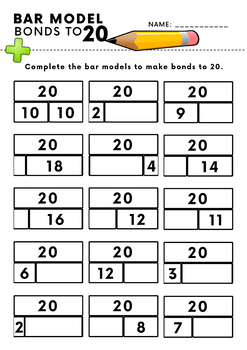digital

# Number Bonds To 20: Bar Model Practise SheetsSubject
Resource Type
Formats

TpT Digital Activity

PDF (11 MB|6 pages)
Standards
\$2.00
TpT Digital Activity
Add notes & annotations through an interactive layer and assign to students via Google Classroom.
\$2.00
TpT Digital Activity
Add notes & annotations through an interactive layer and assign to students via Google Classroom.

### Description

This resource will give your class loads of practise with number bonds to 20 using the bar model method. It’s a non-prep resource which contains three levels of differentiation.

Diff. 1: Complete the bar models by filling in the missing number.

Diff. 2: Complete the bar models by filling in one or two of the missing numbers + a word problem challenge.

Diff. 3: Complete the bar models filling in one or two of the missing numbers + two word problem challenges.

B&W copies are included with this resource.

------------------------

Please don’t forget to rate this product and provide feedback to earn credits that you can use toward future purchases on TpT.

Also be sure to visit my store and FOLLOW ME to see my new products and hear about upcoming sales!

Total Pages
6 pages
N/A
Teaching Duration
N/A
Report this Resource to TpT
Reported resources will be reviewed by our team. Report this resource to let us know if this resource violates TpT’s content guidelines.

### Standards

to see state-specific standards (only available in the US).
Fluently add and subtract within 20 using mental strategies. By end of Grade 2, know from memory all sums of two one-digit numbers.
Add and subtract within 20, demonstrating fluency for addition and subtraction within 10. Use strategies such as counting on; making ten (e.g., 8 + 6 = 8 + 2 + 4 = 10 + 4 = 14); decomposing a number leading to a ten (e.g., 13 - 4 = 13 - 3 - 1 = 10 - 1 = 9); using the relationship between addition and subtraction (e.g., knowing that 8 + 4 = 12, one knows 12 - 8 = 4); and creating equivalent but easier or known sums (e.g., adding 6 + 7 by creating the known equivalent 6 + 6 + 1 = 12 + 1 = 13).
Apply properties of operations as strategies to add and subtract. If 8 + 3 = 11 is known, then 3 + 8 = 11 is also known. (Commutative property of addition.) To add 2 + 6 + 4, the second two numbers can be added to make a ten, so 2 + 6 + 4 = 2 + 10 = 12. (Associative property of addition.)
Use addition and subtraction within 20 to solve word problems involving situations of adding to, taking from, putting together, taking apart, and comparing, with unknowns in all positions, e.g., by using objects, drawings, and equations with a symbol for the unknown number to represent the problem.